# Data Viz - Diagram

A diagram is a visual representation of a graph. It's a chart with arrows between visuals.

A flow chart is a diagram that represents a directed graph

## Tools

Recommended Pages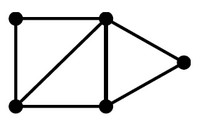(Graph|Network) - Sankey Diagram (Flow)

Sankey diagrams visualize the magnitude of flow between nodes in a network. alluvial diagrams Sankey_diagram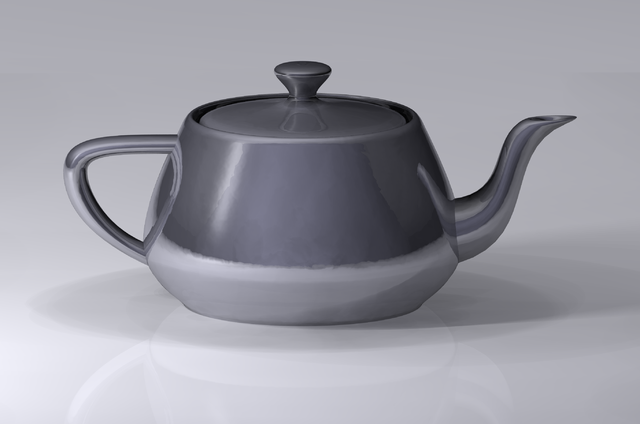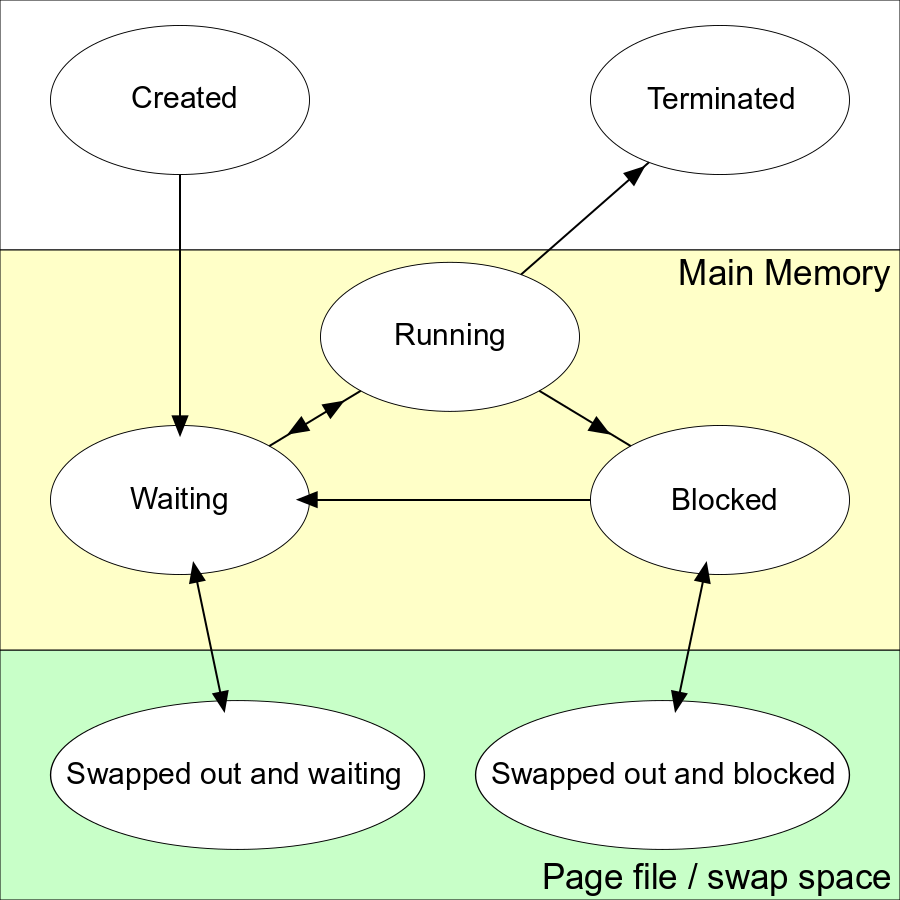Data Visualization - State Diagram

A state diagram is a diagram that visualize the different state of entity (ie lifecycle) The OS process state State_diagramObject - Class Diagram (UML)

A class diagram is a diagram specified in the UML standard by showing: the classes (the class is the entity) with its attributes and methods. and the relationships between them By comparison with...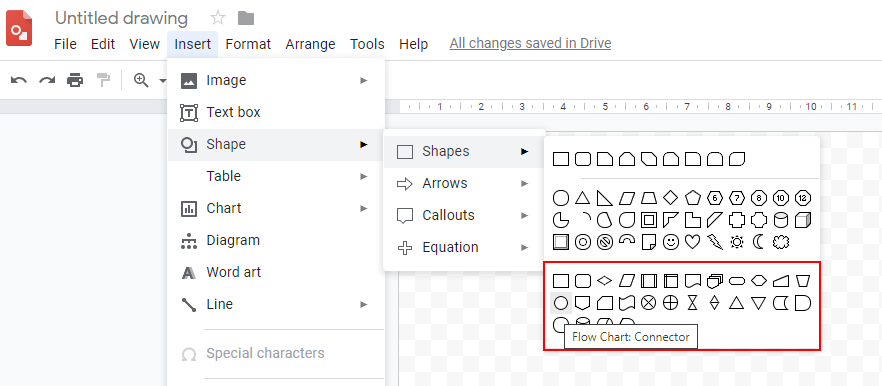Process - Flow Chart

A Flow chart is a diagram that represents a flow with directed graph generally composed of: (vertice (node)) connected by directed lines (edge) The flow passes a material or logical thing from...Process - Sequence Diagram Viz

A Sequence diagram is an interaction diagram that shows how processes operate with one another and in what order. They focuses on the message interchange between participant materialized in a line known...Relational Data Modeling - Entity-Relationship Diagram (ERD)

The entity-relationship (E-R) diagram is a diagram that helps visualize relational data models and shows relationships between relation (ie the entities) ER modeling technique was first developed by Peter...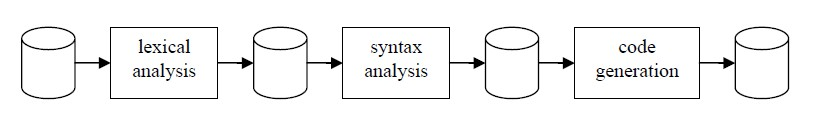A Railroad is a diagram that permits to visualize a grammar. Same as A Railroad diagram is made of: a main diagram a set of syntax diagrams. Each diagram has an entry point and an end point....UML - Interation Diagram

interaction diagram is a type of uml diagram Communication_diagram Unified_Modeling_LanguageUML Interaction diagrams